Dr. J's Maths.com
Where the techniques of Maths
are explained in simple terms.

Trigonometry - Measuring angles - finding the length of sides.
Test Yourself 1.

 Find the length of the side indicated for each question (correct to 1 decimal place). 1.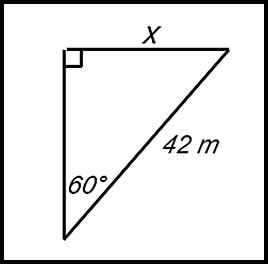Answer.36.4 m. 2.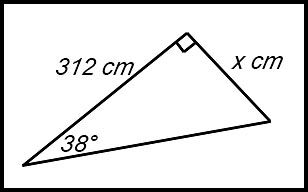Answer.243.8 cm. 3.Answer.19.0 cm. 4.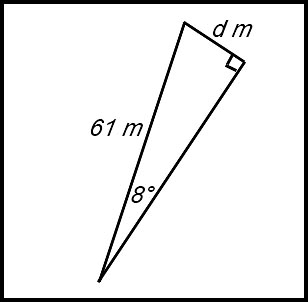Answer.8.5 m. 5. tan 6. cos Find the length of the side indicated for each question (answer correct to 1 decimal place). Note: the pronumeral could be in the denominator. 7.Answer.366.5 m. 8.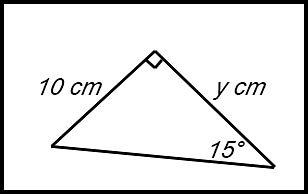Answer.37.3 cm. 9.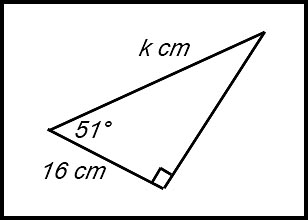Answer.25.4 cm. 10.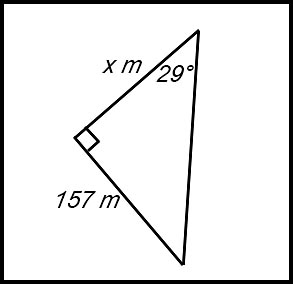Answer.283.2 m. Problems 11. A 3 metre ladder is leaning against a wall. The angle between the ladder and the floor is 55°. (i) How far does the ladder reach up the wall (nearest cm)? (ii) How far is the foot of the ladder away from the wall (nearest cm)? Answer.(i) Up the wall: 246 cm.(ii) From the wall: 172 cm.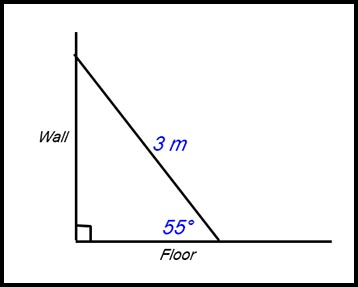12. 13. 14. probs denom